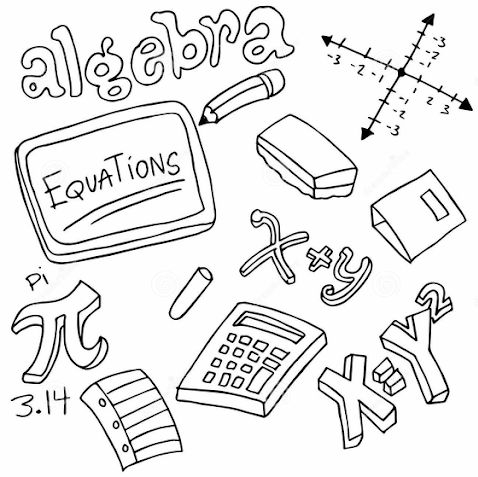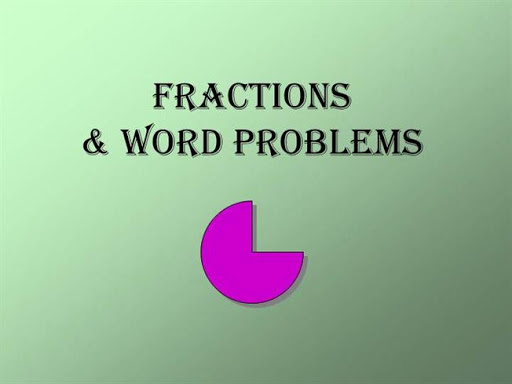# Word problems on algebraic fraction

Welcome to Class !!

In today’s Mathematics class, We will be discussing Algebraic Fractions. We hope you enjoy the class!

CONTENTS

• From words to algebra
• Word problems with brackets
• Word problems with fractions.
• From words to AlgebraWhen solving word problems:

1. Choose a letter for the unknown
2. Write the information of the equation in algebraic form
3. Make an equation
4. Solve the equation
5. Give the answer in written form.
6. check the results against the information given in the question.

Example:

1. I think of a number. Multiply it by 5 and add 15. The result is 100. What is the number I thought of?

Let the number be n

I multiply n by 5:  5n

I add 15: 5n + 15

The result is 100: 5n + 15 = 100 ………..   ( i)

Subtract 15 from both sides

5n + 15 -15 = 100 – 15

5n = 85 ……………………………….  2

Divide both sides by 5

n = 17

Check:  17 x 5 = 85 + 15 = 100

EVALUATION

1. John thinks of a number, he doubles it. His result is 58.  What number does he think of?
2. A number is multiplied by 6 and then 4 is added. The result is 34.  Find the number.

Example

A rectangle is 8m long and its perimeter is 30m. Find the breadth of the rectangle.

Solution

Let the breadth of the rectangle be “b” meters.

Perimeter = 8 + b + 8 + b meters

= 16  + 2b = 30

Subtract 16 from both sides

2b = 30 -16 = 14

Divide both sides by 2

B = $\frac{14}{2}$  = 7

The breadth of the rectangle is 7 meters

Check: 8m + 7m + 8 + 7m = 30m

EVALUATION

1. A rectangle is 10m long and its perimeter is 26m. Find the breadth of the rectangle.
2. A square has a perimeter of 32m. Find the length of one side of the square.

#### Word problems with brackets.

Example

I subtract 3 from a certain number, multiply the result by 5 and then add 9.  If the final result is 54, find the original number.

Solution

Let the original number be x.

I subtract 3, this gives x – 3.

I multiply by 5 this gives 5(x- 3)

I add 9 this gives 5 (x – 3) + 9

The result is 54.

So, 5 ( x – 3) + 9 = 54 ………..1

Clear brackets

5x – 15 + 9 = 54……………….2

Collect like terms

5x = 54 + 15 – 9 =60

x = 60 ÷ 5 = 12

The original number is 12

Evaluation

1. I subtract 8 from a certain number. I then multiply the result by 3. The final answer is 21. Find the original number.
2. I thought of a number, I multiplied it by 5. I then subtracted 19. Finally, I doubled the result, the result was 22. What number did I think of?

Worked Examples

Find two consecutive even number such that seven times the smaller number subtracted from nine times the greater number makes 46.

Solution

Note : 1, 2, 3, 4,5 ……………..are consecutive whole numbers

2,4,6,8,10 …………..are consecutive even numbers

1,3,4,7,9…………………….are consecutive odd numbers.

Let the number be x and (x + 2)

Multiply  x by 7, gives 7x

Multiply (x + 2) by 9 gives 9 (x + 2)

The result is 46.

9(x+2) – 7x = 46 …………………….1

9x + 18 – 7x = 46 ……………………2

9x – 7x = 46 – 18 ……………………..3

2x = 28 …………………………………4

X = 28/2 = 14

If x = 14

(x+ 2) = 14 + 2 = 16

The numbers are 14 and 16.

EVALUATION

1. Find the consecutive whole numbers such that five times the smaller number added to three times greater number makes 59. (Hint: let the numbers be x and (x +1).
2. Find two consecutive odd numbers such that six times the smaller added to four times the greater comes to 138. (Hint: let the number is x and x + 2)##### Word problems with Fractions

Examples: I add 55 to a certain number and then divide the sum by 3.  The result is four times the first number, find the number.

Let the number be n

I add 55 to n this gives n + 55

I divide the sum by 3: this gives $\frac{n+55}{3}$

The result is 4n

So,  ………………..1

Multiply both sides by 3

…………………..2

n + 55 = 12 n ………………………………3

Collect terms

55 = 12n – n

55 = 11n

So, n = 5

EVALUATION

I think of a number. I double it. I divide the result by 5. My answer is 6. What number did I think of?

New General Mathematics, UBE Edition chapter 13 pgs 114-118

Essential Mathematics by AJS Oluwasanmi, chapter 19pgs 205-207

GENERAL EVALUATION

1. A woman is 7 times as old as her daughter in 2 years’ time, she will be 5 times as old as her daughter. How old are they now?
2. If 60 is added to a number, and the sum is divided by 3, the result is 7 times the same number. Find the number.

REVISION QUESTION

1. I think of a number. I add 7  to it and then divide the sum by 4. The result is 5. What is the number?
2. A man spent two-third of his monthly wages on his car and one quarter on food. If he spent #5500 altogether, how much does earn in a  month?
3. If 18  is added to a  number to a  number,  and the sum is divided by  2, the result is  5 times the number. Find the number.

New General Mathematics pg. 209-211

WEEKEND ASSIGNMENT

1. What is the perimeter of an equilateral triangle of side 2b meters? A. 4b B. 5b C. 6b D. 8b
2. How many altogether if a number is 3 less than a? A. a -3 B. – a + 3 C. 3 – a D. 3 + a
3. How many altogether if a number is doubled and then 5 is taken away? A. 5 – 2y B. 2y – 5 C. 5y – 2 D. 2 – 5y
4. What is the perimeter of a rectangle of breadth b meters and length three times as long? A. 4b meters B. 5bmeters   C. 8b meters          D. 10bmeters
5. What is the perimeter of a rectangle of length y meters and breadth 4m less than the length? A. 4(y -2) meters B. 2 (y- 4)meters      C. 4 (2-y)       D. 2 (4- y)meter

THEORY

1. A rectangle is such that its breadth is 2m less than its length. Find the length of the rectangle if the perimeter is 8m.
2. I am thinking of a number. I take away 5, the result is 14. What number did it think of?

We have come to the end of this class. We do hope you enjoyed the class?

Should you have any further question, feel free to ask in the comment section below and trust us to respond as soon as possible.

In our next class, we will be talking about Linear Inequalities. We are very much eager to meet you there.

For more class notes, videos, homework help etc download our Mobile App HERE

Join ClassNotes.ng Telegram Community HERE for exclusive content and support

### 1 thought on “Word problems on algebraic fraction           ”

1.preciousndubuisih

Please can you help me with my assignment that are difficult

Don`t copy text!